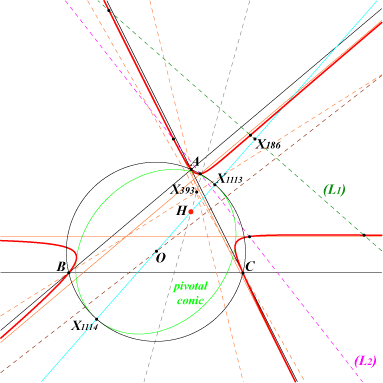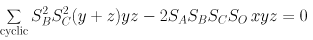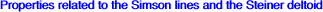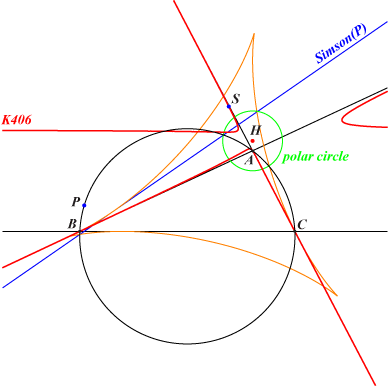X(4), X(877), X(4240)K406 is a member of the class CL046. Let (H) be a rectangular circum-hyperbola and (N) its normal at H. (N) meets (H) at H and another point M which lies on K406. K406 is the cK with pole X(393), root X(2), singularity X(4) where X(393) is the barycentric square of H. K406 is the H-isoconjugate of K010, see below. It is also the barycentric product of K010 by H. K406 has three real asymptotes parallel to the sidelines of ABC forming a triangle homothetic of ABC under the homothety with center X(393) and ratio 2 cotA cotB cotC cotw, where w is the Brocard angle. K406 meets its asymptotes on (L2), a perpendicular to the Brocard axis. The real inflexion points lie on (L1), the perpendicular at X(186) to the Euler line. Recall that X(186) is the inverse of H in the circumcircle. The pivotal conic is inscribed in the antimedial triangle and in the anticevian triangle of H. Its center is O and it passes through X(1113), X(1114), the intersections of the Euler line and the circumcircle. Thus, the circumcircle is the principal circle of the conic. Its foci are H and L=X(20) and its excentricity is OH/R. Hence, its is an ellipse when ABC is acute angle. Note that (L1) is the directrix relative to the focus H. This conic is the MacBeath conic of the antimedial triangle. See also the generalization in the page K052. See here for a property by Angel Montesdeoca, in Spanish, 2023-04-13.written with the collaboration of Dominique Roux K406 is the reciprocal polar transform of the Steiner deltoid H3 with respect to the polar circle. In other words, the pole S of the Simson line of a point P (on the circumcircle) with respect to the polar circle is a point on K406. Recall that the trilinear pole T of the Simson line of P lies on K010. Note that S and T are H-isoconjugate points i.e. their barycentric product is H. *** Let (L) be a line passing through H. This can be seen as the Steiner line of a point Q on (O) which is the antipode of the isogonal conjugate of the infinite point of (L). The perpendicular at H to (L) meets the Simson line of Q at a point whose inverse R in the polar circle lies on K406. R is the orthoassociate of Q. Generalization The polar circle can be replaced by any diagonal conic D(M = u:v:w) with an equation of the form : x^2/u + y^2/v + z^2/w = 0. The reciprocal polar transform of the Steiner deltoid H3 with respect to this diagonal conic D(M) is the conico-pivotal cubic K(M) = cK(#M, X69 x M) = nK(M^2, X69 x M, M) where X69 x M denotes a barycentric product. This cubic is always an acnodal cubic with node M. • The pivotal conic is inscribed in the anticevian triangle of M with perspector X194 x M. • The contact conic is the circum-conic with perspector X5 x M. • The three points of inflexion lie on the trilinear polar of M ÷ X5 (barycentric quotient). With M = X2, X6, X4 we obtain the cubics K010, K162, K406 respectively. Special case : M lies on the line at infinity D(M) is a parabola inscribed in the medial triangle. This is the complement of the parabola inscribed in ABC whose perspector is the isotomic conjugate of M. The third point at infinity of K(M) is that of the direction perpendicular to M. • With M = X(512) we obtain cK(#X512, X647) passing through X(511), X(512), X(1495), X(2028), X(2029). • With M = X(513) we obtain cK(#X513, X905) passing through X(513), X(517), X(910), X(1455), X(2446), X(2447). • With M = X(523) we obtain K741 = cK(#X523, X525) which is probably the most interesting case. In all cases, – the root of the cubic K(M) lies on the trilinear polar of X(69), a line passing through X(441), X(525), X(647), X(905), X(2504), X(2510), X(2519), X(2522), X(2523), X(2524), X(2525). – the pivotal conic and the contact conic meet at a point lying on the Euler line of the medial triangle and three other points lying on the axes of H3. The triangle formed by these three points is equilateral if and only if M = X(523) corresponding to the cubic K741. This is the only case when the two conics are rectangular hyperbolas. – the inflexions of K(M) lie on the sidelines of the equilateral triangle which is the image of the CircumTangential triangle under the translation that maps X(3) onto X(5). The envelope of the line passing through these inflexions is the inconic with perspector X(95) and center X(140).